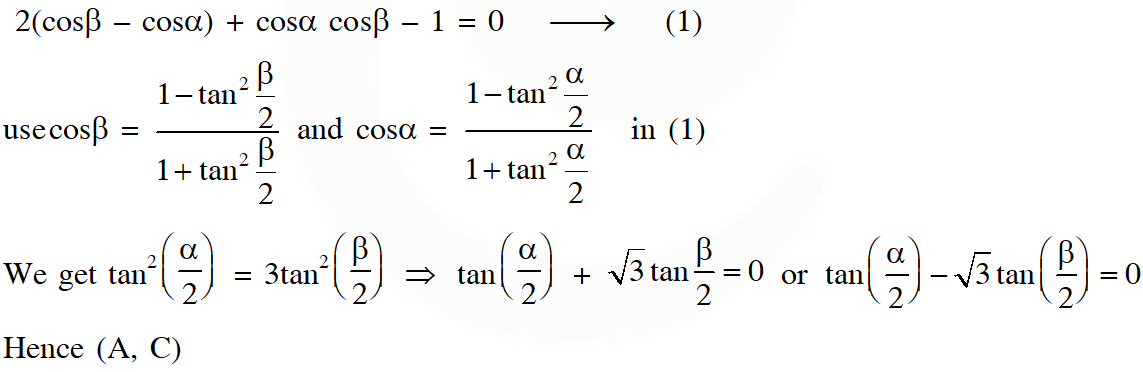Most Affordable JEE | NEET | 8,9,10 Preparation by Kota's Top IITian Doctor Faculties

# Compound Angle - JEE Advanced Previous Year Questions with Solutions`
JEE Advanced Previous Year Questions of Math with Solutions are available at eSaral. Practicing JEE Advanced Previous Year Papers Questions of mathematics will help the JEE aspirants in realizing the question pattern as well as help in analyzing weak & strong areas. eSaral helps the students in clearing and understanding each topic in a better way. eSaral also provides complete chapter-wise notes of Class 11th and 12th both for all subjects. Besides this, eSaral also offers NCERT Solutions, Previous year questions for JEE Main and Advance, Practice questions, Test Series for JEE Main, JEE Advanced and NEET, Important questions of Physics, Chemistry, Math, and Biology and many more. Download eSaral app for free study material and video tutorials. Simulator Previous Years JEE Advance Questions One or more than one is/are correct : [Q.1(a) & (b)]
Q. If $\frac{\sin ^{4} x}{2}+\frac{\cos ^{4} x}{3}=\frac{1}{5},$ then (A) $\tan ^{2} x=\frac{2}{3}$ (B) $\frac{\sin ^{8} x}{8}+\frac{\cos ^{8} x}{27}=\frac{1}{125}$ (C) $\tan ^{2} \mathrm{x}=\frac{1}{3}$ (D) $\frac{\sin ^{8} x}{8}+\frac{\cos ^{8} x}{27}=\frac{2}{125}$ [JEE 2009, 4 + 4]
Ans. (A,B) (a) $\frac{\tan ^{4} x}{2}+\frac{1}{3}=\frac{\sec ^{4} x}{5}$ put $\tan ^{2} \mathrm{x}=\mathrm{t}$ on solving we get $t=2 / 3$ $\Rightarrow \sin ^{2} x=\frac{2}{5} \quad \Rightarrow \quad \cos ^{2} x=\frac{3}{5}$
Q. For $0<\theta<\frac{\pi}{2},$ the solution(s) of $\sum_{m=1}^{6} \csc \left(\theta+\frac{(m-1) \pi}{4}\right) \csc \left(\theta+\frac{m \pi}{4}\right)=4 \sqrt{2}$ is (are) (A) $\frac{\pi}{4}$ (B) $\frac{\pi}{6}$ (C) $\frac{\pi}{12}$ (D) $\frac{5 \pi}{12}$ [JEE 2009, 4 + 4]
Ans. (C,D)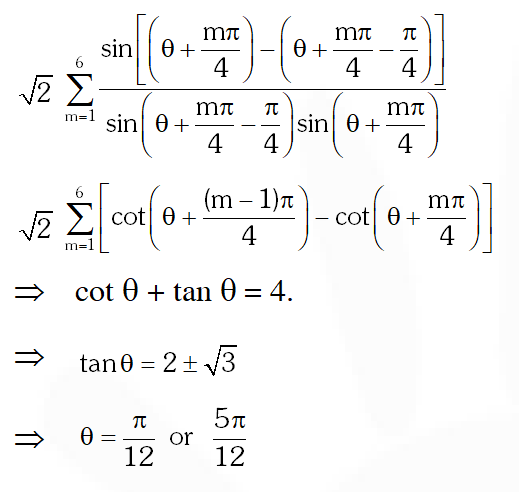Q. The maximum value of the expression $\frac{1}{\sin ^{2} \theta+3 \sin \theta \cos \theta+5 \cos ^{2} \theta}$ is [JEE 2010,3+3]
Ans. 2
Q. Two parallel chords of a circle of radius 2 are at a distance $\sqrt{3}+1$ apart. If the chords subtend at the center, angles of $\frac{\pi}{k}$ and $\frac{2 \pi}{k}$ where $\mathrm{k}>0$, Glue of $[\mathrm{k}]$ is - [JEE 2010,3+3]
Ans. k=3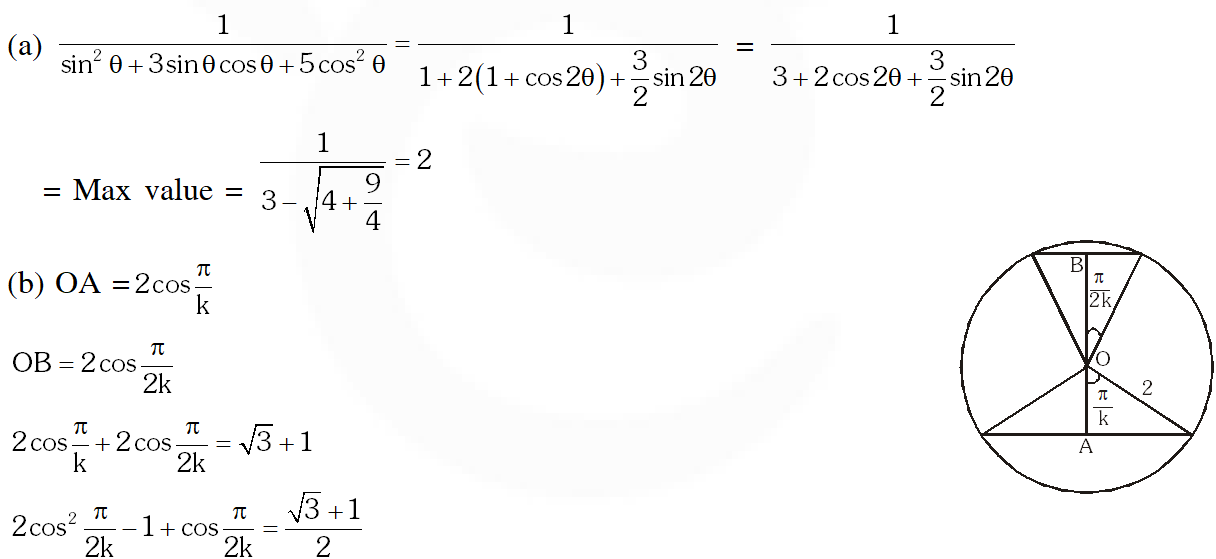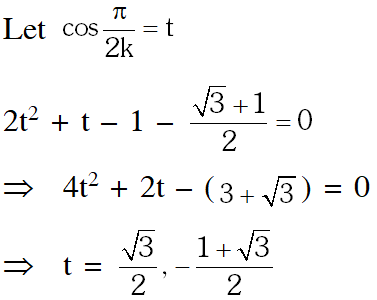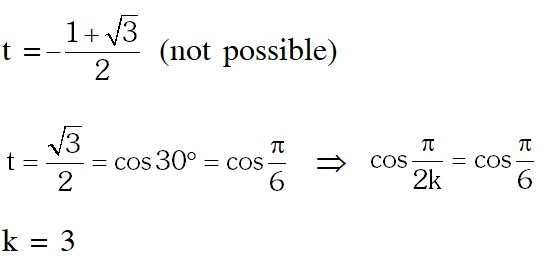Q. Let $\mathrm{P}=\{\theta: \sin \theta-\cos \theta=\sqrt{2} \cos \theta\}$ and $\mathrm{Q}=\{\theta: \sin \theta+\cos \theta=\sqrt{2} \sin \theta\}$ be two sets. Then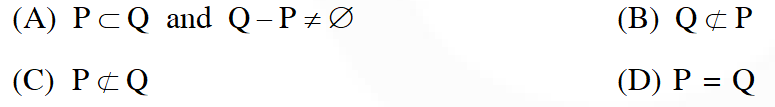[JEE 2011,3]
Ans. (D)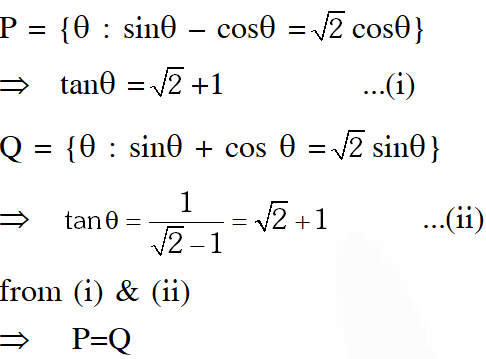Q. The value of $\sum_{\mathrm{k}=1}^{13} \frac{1}{\sin \left(\frac{\pi}{4}+\frac{(\mathrm{k}-1) \pi}{6}\right) \sin \left(\frac{\pi}{4}+\frac{\mathrm{k} \pi}{6}\right)}$ is equal to (A) $3-\sqrt{3}$ (B) $2(3-\sqrt{3})$ (C) $2(\sqrt{3}-1)$ (D) $2(2+\sqrt{3})$ [JEE Advance 2016]
Ans. (C)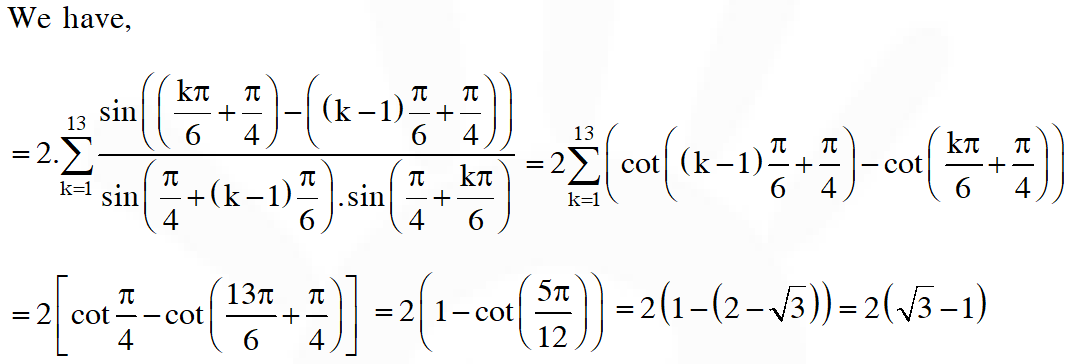Q. Let $\alpha$ and $\beta$ be nonzero real numbers such that $2(\cos \beta-\cos \alpha)+\cos \alpha \cos \beta=1$. Then which of the following is/are true ? (A) $\tan \left(\frac{\alpha}{2}\right)-\sqrt{3} \tan \left(\frac{\beta}{2}\right)=0$ (B) $\sqrt{3} \tan \left(\frac{\alpha}{2}\right)-\tan \left(\frac{\beta}{2}\right)=0$ (C) $\tan \left(\frac{\alpha}{2}\right)+\sqrt{3} \tan \left(\frac{\beta}{2}\right)=0$ (D) $\sqrt{3} \tan \left(\frac{\alpha}{2}\right)+\tan \left(\frac{\beta}{2}\right)=0$ [JEE Advance 2017]
Ans. (A,C)